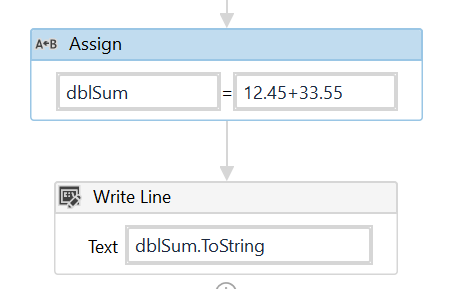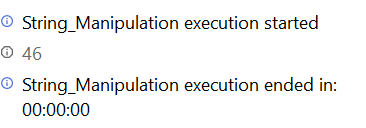# How to add sum of values in single variable

How to add values to variables .eg value 12.45+12.45 stored in single varaible eg:cash value=12.45+33.55

@sruthesanju - Try like this…dblsum is of variable type Double

Output:Cahvalue=“12.45+22.45” how to add this.this "12.45+22.45"value I will get as input which is stored in one variable not in two variables and stored in cashvalue which is string type

Hi try like this @sruthesanju

Split(Cahvalue,“+”).Sum(Function(e) CDbl(e)). ToString in writeline activitiy

You will get the sum of the numbers directly

Regards

Nived NHappy Automation2 Likes

What if I get input as alternative symbol like12.34-34.56+67.8

@sruthesanju Can you tell us what are the different Arithmetic operations and How many maximum operands can be present in your input Expression ?

It will have addition and subtraction alone

@sruthesanju Taking into Consideration that the requirement is only for Addition and Subtraction, we may be able to minimise the steps required by matching the numbers using Regex and then taking the Total Sum.

Check the below example workflow :
Test it with all possible inputs that you would get to make sure it works properly.
Regex Find.zip (1.6 KB)

Let us know if it was not the expected solution.

hello

part of my project is to sum two numbers that i will take from a website
so, i used the get text and i put each one of them into a variable and i used the method that you mentioned but it does not sum the number because they are in form of text

how to convert them so i can sum them and is there any other method that i can use for the project that i work on ???